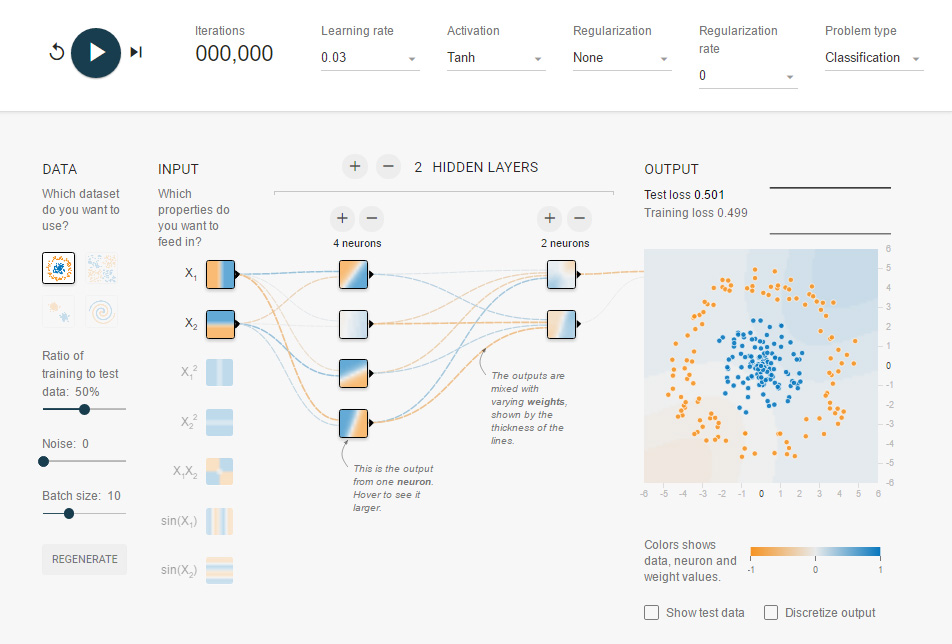### Tensor Calculations with the Eigen C++ Library#### Course Content:

This course is an introduction to Tensor calculations with Eigen, a popular C++ library for working with numerical arrays and linear algebra. It covers the following topics:

• We learn the concept and techniques of the Eigen Tensor class
• How to declare, initialize Tensors of various ranks and types and how to access Tensor elements
• Elementary unary and binary operations involving Tensors
• More complex operations (reductions, contractions)
• Modifying the shape of Tensors

#### Who Is This Course For:

Developers in any Domain that need to use higher-dimensional numerical data containers

• Statistical Calculations
• Machine Learning

#### How Does The Course Help:

Eigen is a fairly large library. The course aims to:

• Introduce the Tensor part of the library and its purpose
• Sketch its overall structure and functionality
• Familiarize with the common usage patterns (API's)

#### What Will You Get From The Course:

• You will be able to confidently use Eigen::Tensor to solve common numerical processing tasks, in particular those requiring standard manipulation of tensors
• You will be able to contribute to the specific use cases mentioned above

#### Course Level and Difficulty Level:

This course is part of the Data Science family.

• This is a Core Level course in Data Science, which means that good grounding at Introductory level to various Data Science topics is a prerequisite for making the most out of this course.
• This is a Technical course which means certain mathematical (linear algebra) and/or technology elements (C++) are assumed as known before one can master the course material.

#### Advanced material not covered here:

• Memory layouts (how numerical data are stored in memory) and the performance implications
• Extending Eigen (in particular the C-API's)
• Numerical algebra / scientific computing concepts beyond what is needed to understand the core Eigen::Tensor functionality

If you have not taken an Open Risk Academy course before the "CrashCourse Academy Demo" provides a quick overview of the Academy.

The following table places the course in the Open Risk skills diagram:

 Introductory Level Core Level Advanced Level Non-technical Technical DAT31071

#### Course Material:

The course material comprises the following: